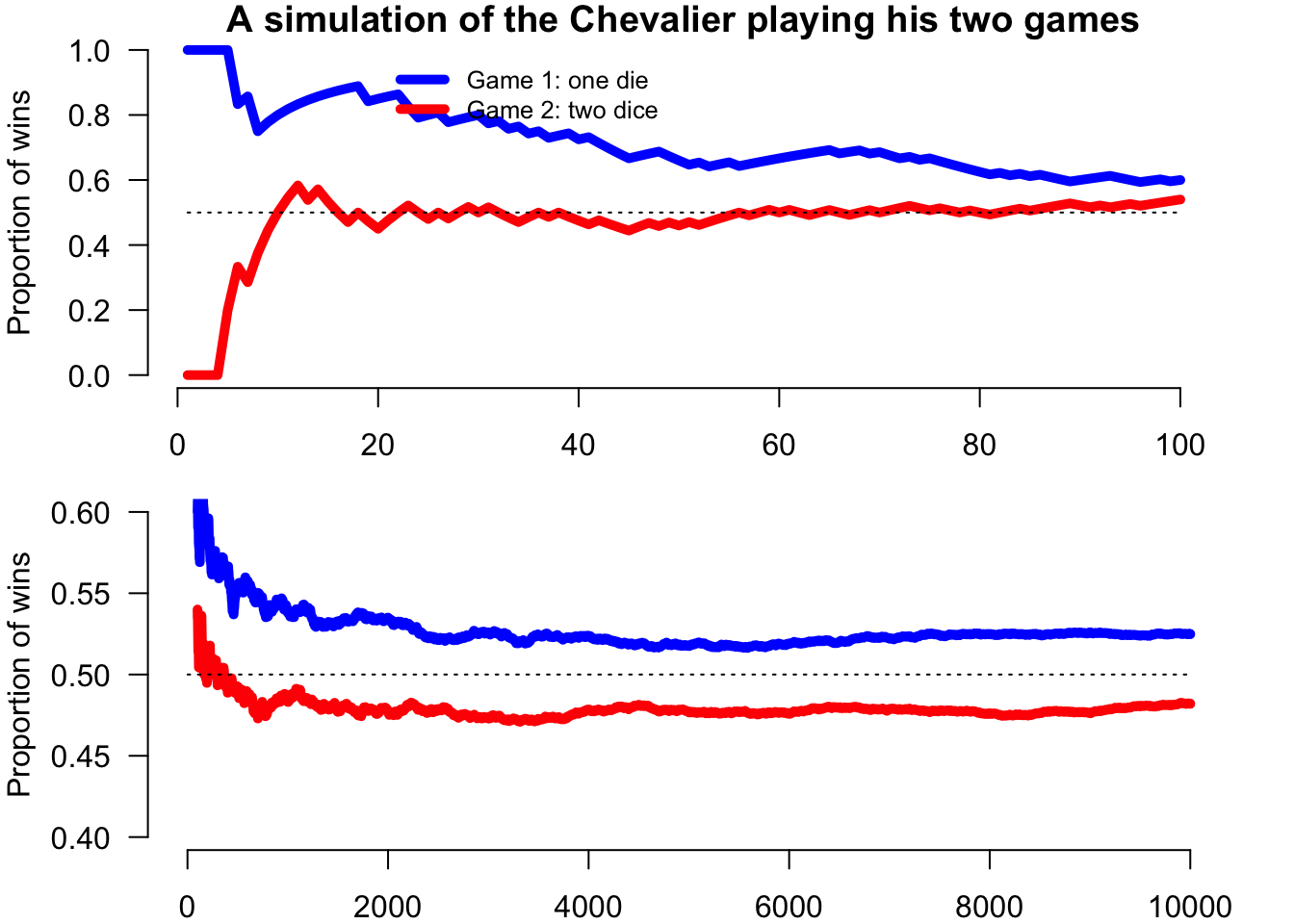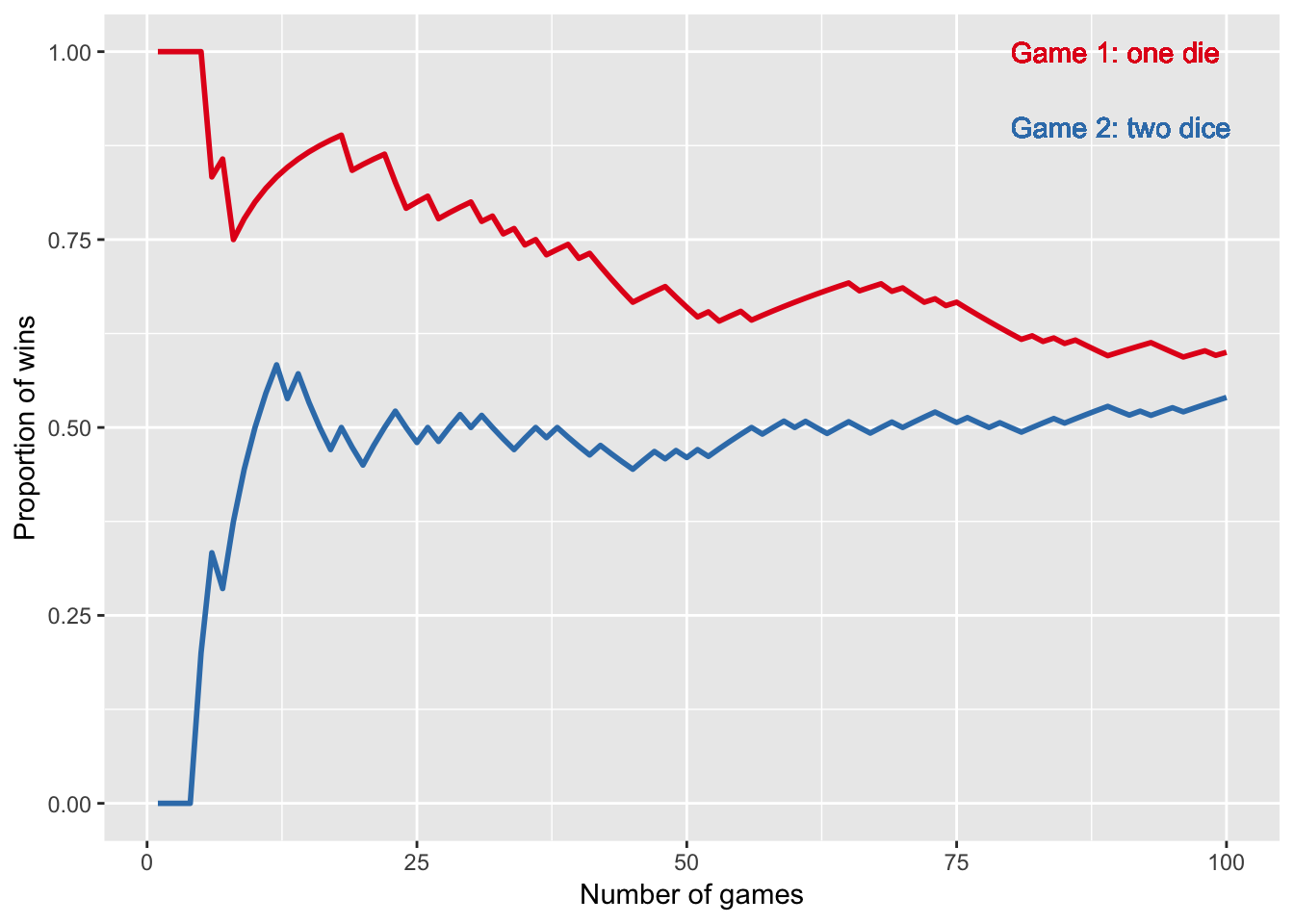The data is simulated within the code, and so will be different every time - this code will not produce the plots in the book, as I forgot to save the random-number seed.

nsims=10000
n=1:nsims
sixes1<-rbinom(nsims,4,1/6)  # simulate the number of sixes in 4 throws of one dice
sixes2<-rbinom(nsims,24,1/36) # simulate the number of six-pairs in 24 throws of two dice
game1=cumsum(sixes1>0)/(1:nsims) # proportion of 'successes'
game2=cumsum(sixes2>0)/(1:nsims)
df=cbind.data.frame(n,game1,game2)

First in R base graphics

par(mfrow=c(2,1),mar=c(2,4,1,2))
nsim1=100
plot(1:nsim1, game1[1:nsim1], type="n",xlab="number of games",ylab="Proportion of wins",
ylim=c(0,1.0),axes=F,      main="A simulation of the Chevalier playing his two games")
axis(1) #,at=(seq(0,100,by=5)))
axis(2, las=1 )
lines(1:nsim1,game1[1:nsim1],lty=1, lwd=5,col="blue")
lines(1:nsim1,game2[1:nsim1],lty=1, lwd=5,col="red")
lines(c(1,nsim1),c(0.5,0.5),lty=3,lwd=1)
legend(20,1,bty="n",col=c("blue","red"),legend=c("Game 1: one die","Game 2: two dice"),lwd=5, cex=0.8)

plot(nsim1:nsims, type="n",xlab="number of games",ylab="Proportion of wins",
ylim=c(0.4,0.6),axes=F,      main="")
axis(1) #,at=(seq(0,100,by=5)))
axis(2, las=1 )
lines(nsim1:nsims,game1[nsim1:nsims],lty=1, lwd=5,col="blue")
lines(nsim1:nsims,game2[nsim1:nsims],lty=1, lwd=5,col="red")
lines(c(1,nsims),c(0.5,0.5),lty=3,lwd=1)Figure 8.1. A computer simulation of 10,000 repeats of two games. In Game 1, you win if you throw a six in at most 4 throws of a fair die; in Game 2, you win if you throw a double-six in at most 24 throws of two fair dice. Over the first 100 games of each (upper chart) it may sometimes look like there is a higher chance of winning Game 2, but after thousands of plays (lower chart) it becomes apparent that Game 1 provides a marginally better bet.

Now in ggplot

library(ggplot2)
P <- list() # prepare list for the plots
for(i in 1:2) # 2 runs with parameter i
{
p <- ggplot(head(df,100^i), aes(x=n, y))# + xlim(0,100^i) # create initial plot from dataframe restricting to first 100 data-points if i=1 (otherwise uses the full 10000 data-points)
p <- p + geom_line(aes(y=game1, col="Game 1"), size=1) # add game 1 series
p <- p + geom_line(aes(y=game2, col="Game 2"), size=1) # add game 2 series

# add custom legend only on first run
if (i==1) {p <- p + geom_text(aes(x = 80, y = 1, label = "Game 1: one die", color = "Game 1", hjust=0))
p <- p + geom_text(aes(x = 80, y = 0.9, label = "Game 2: two dice", color = "Game 2", hjust=0))}
p <- p +labs(x="Number of games", y="Proportion of wins") # Adds title, subtitle, and caption
p <- p + scale_colour_brewer(palette = "Set1") # sets the colour palette
p <- p + theme(legend.position="none")#, legend.box = "horizontal") # removes the legend
p <- p + theme(plot.caption=element_text(hjust = 0.5)) # centre justifies any caption
P <- c(P, list(p)) # add plot to the plot-list
}
# draw the plots

p <- P
p
## []p <- P
p
## []